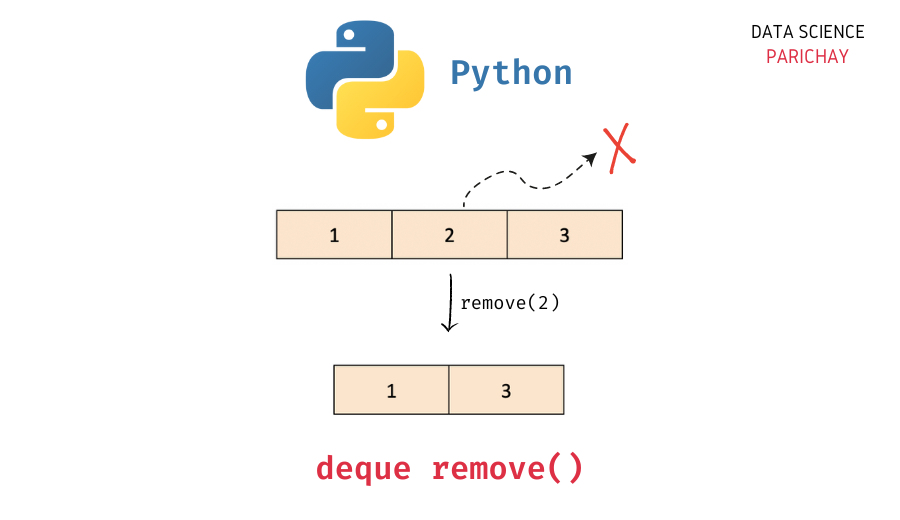# Python – Remove Element by Value in Deque

In this tutorial, we will look at how to remove an element by value from a deque in Python with the help of some examples.

## `deque` in Python

The `collections` module in Python comes with a `deque` class that you can use to implement a stack or queue data structure in Python. Deques support thread-safe, memory efficient appends and pops from either side of the deque with approximately the same O(1) performance in either direction.

In simple terms, you can think of a `deque` in Python as a list-like data structure but with efficient insertion and removal of items from either end (left and right). This makes them very useful when implementing a stack or queue functionality in Python.

## How to remove an element by value from a deque in Python?

The `deque` class comes with a number of built-in functions to help perform common operations (like adding and removing elements).

To remove an element by value from a deque in Python, use the deque `remove()` function. Pass the element you want to remove as an argument. The following is the syntax –

```# remove element "e" from deque "queue"
queue.remove(e)```

It removes the first occurrence of the element `e` in the deque.

## Examples

Let’s now look at some examples of using the above syntax –

### Example 1 – Remove an element by value from a deque

First, let’s create a deque with some elements.

📚 Data Science Programs By Skill Level

Introductory

Intermediate ⭐⭐⭐

🔎 Find Data Science Programs 👨‍💻 111,889 already enrolled

Disclaimer: Data Science Parichay is reader supported. When you purchase a course through a link on this site, we may earn a small commission at no additional cost to you. Earned commissions help support this website and its team of writers.

```from collections import deque

# create a deque
queue = deque([1, 2, 3])
# print the deque
print(queue)```

Output:

`deque([1, 2, 3])`

Here, we imported the `deque` class from the `collections` module and created a deque object, `queue` with three elements – 1, 2, and 3.

Now, let’s now remove the element `2` from the deque created above.

```# remove element 2 from deque
queue.remove(2)
# print the deque
print(queue)```

Output:

`deque([1, 3])`

Here, we used the deque `remove()` function to remove all the element `2` from the deque `queue`.

What happens if there are multiple elements with the same value (that you want to delete)?

In that case, the deque `remove()` function removes the first occurrence of the value inside the deque.

Let’s look at an example.

```# create a deque
queue = deque([1, 2, 3, 2, 4])
# remove element 2 from deque
queue.remove(2)
# print the deque
print(queue)```

Output:

`deque([1, 3, 2, 4])`

Here, the deque `queue` contains the element `2` at two different indices. When we use the deque `remove()` function and pass `2`, the first occurrence of the element is removed from the deque.

To remove all the occurrences of an element from a deque, use the `remove()` inside a `while` loop like below.

```# create a deque
queue = deque([1, 2, 3, 2, 4])
# remove all occurrences of a value from deque
val = 2
while val in queue:
queue.remove(val)
# print the deque
print(queue)```

Output:

`deque([1, 3, 4])`

All the occurrences of the element `2` is removed.

### Example 2 – Remove an element not present in the deque

Let’s now try to remove an element that is not present in a deque.

```# create a deque
queue = deque([1, 2, 3])
# remove element 4 from deque
queue.remove(4)
# print the deque
print(queue)```

Output:

```---------------------------------------------------------------------------
ValueError                                Traceback (most recent call last)
Input In , in <module>
2 queue = deque([1, 2, 3])
3 # remove element 4 from deque
----> 4 queue.remove(4)
5 # print the deque
6 print(queue)

ValueError: deque.remove(x): x not in deque```

Here, we created a deque with elements 1, 2, and 3 and then used the `remove()` function to remove the element 4 (which is not present in the deque). Since the element is not in the deque, we get a `ValueError`.

You might also be interested in –

•